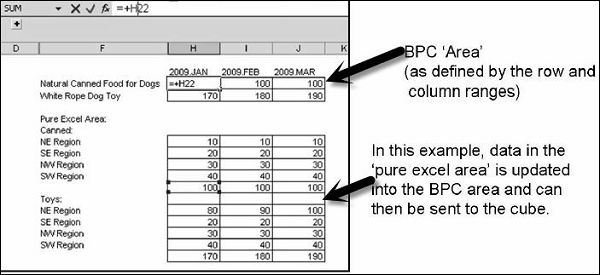# SAP BPC - Business Logic

You can perform business logic and script logic on the data in BPC. There are various calculations that can be performed.

Business Rules − These are monetary functions which are used to post common accounting tasks in Business Planning and Consolidation model.

Script Logic − This includes the formulas which are used to perform different calculations on the members, dimensions, and data in BPC system.

## Dimension Logic

Dimension logic is used to apply calculations and formulas on the dimensions. Calculations are performed as per the dimension formula at retrieval time. It is important to use the dimension formulas correctly as they can affect the system performance if used incorrectly.

Key points to remember while applying Dimension logic

• Dimension formula affects the system performance so they should be applied correctly.

• Dimension formulas should be applied only on the members for which calculations are required after the aggregation.

• Dimension formulas should be used only for those ratios which has to be calculated at both the levels - Base and Parent Level.

• Common Dimension member formulas are used to perform calculations - costs per unit, growth rates, etc.

## How to Define Dimension Logic?

You should be familiar with Multi Dimension Expression language to create complex Dimension formulas. The list of supported MDX functions is available from functional module BAPI_MDPROVIDER_GET_FUNCTIONS.

Prerequisites − To use a formula in dimension, you should add a property called Formula to member sheets using manage dimension task option.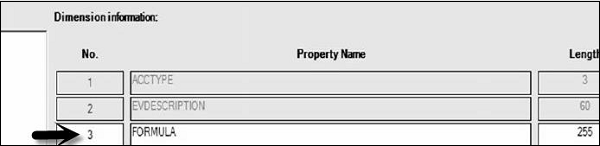You should also define the length of field and it should be more than 60 characters. Higher field lengths are preferred as any length greater than 60 is stored as string in SAP NetWeaver.

If you want to use Dimension formula in AppSet then you should maintain a special dimension member of type R. This member is called as Local Currency (LC).

App set → Select Maintain Dimension Members.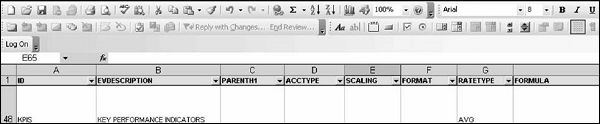The formula can be manually created or taken from a library dimension formula. To define member dimension formula, you have to put a Square bracket around the dimension and there is a dot between the two.

[PRODUCT].[PRODUCT1] – [PRODUCT].[PRODUCT2]

[SALES].[SALES1] / [SALES].[SALES2]

Standard Arithmetic functions has to be used in dimension formula - addition (+), subtraction (−), multiplication (*), Division (/) - these are used in SAP NetWeaver.

Once you save the dimension formulas, next is to validate the syntax.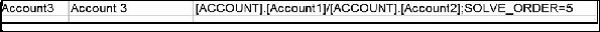## Logic Scripts

Logic scripts allow you to automate the calculations in BPC for the financial statements.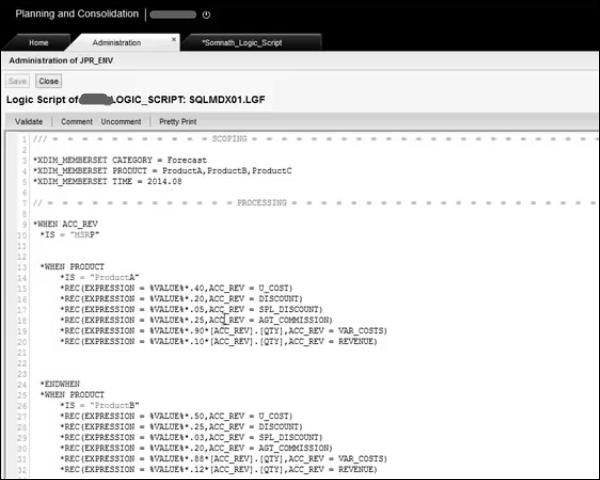Business rules are used to customize the data manipulation task like bulk data imports with currency translations, input data to database, etc. Business rules can be modified using table-based logic or scripts-based filters. Table-based logic provides the features available in UCON accelerator and Script-based files can be customized using MDX or SQL syntax.

You can define business rules for the following activities −

• Carry forward rules − Includes initializing balances when a new fiscal year starts.

• Validation rules − Validates the input data.

• Currency conversion rules − Converts the local currencies into the currency used in BPC reporting.

• Intercompany booking rules − Matches intercompany transactions.

• Automatic Adjustments and Other Calculations.

To manage business rules in BPC system − Go to Administration as shown in the following screenshot. Go to Rules → Expand → Business Rules.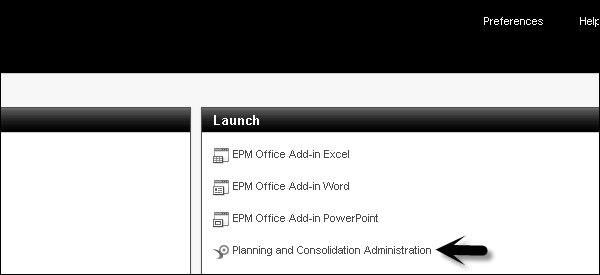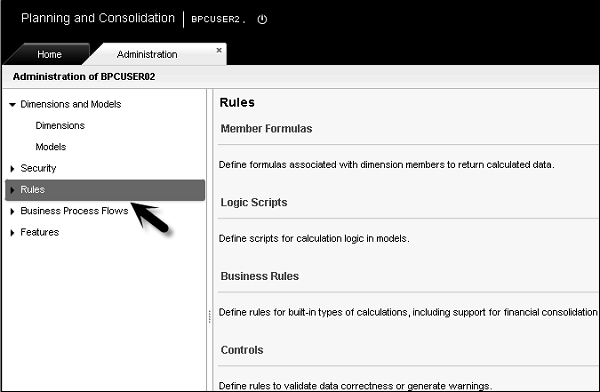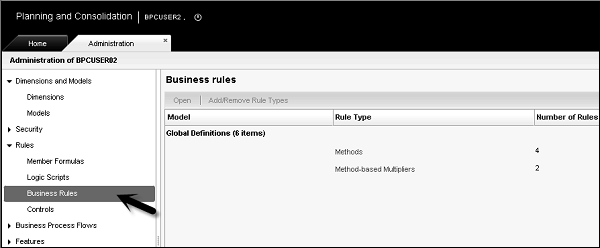## Script Logic

With help of script logic, you can write your own logic in SQL or MDX language. There are various MDX functions that can be used in dimension formulas. Some of the common MDX functions are −

• Avg − Returns average value of a numeric expression.

• Count − Returns the number of tuples in one set and also includes null cells.

• Max − Returns the maximum value of a numeric expression.

• Min − Returns the minimum value of a numeric expression.

• Sum − Returns the sum of numeric expression.

There are other MDX functions that can be used in dimension formulas.

Examples of simple rule formulas

[PRODUCT].[PRODUCT1] – [PRODUCT].[PRODUCT2]

[SALES].[SALES1] / [SALES].[SALES2]

Standard Arithmetic functions have to be used in dimension formula - addition (+), subtraction (−), multiplication (*), Division (/) - these are used in SAP NetWeaver.

Once you save the dimension formulas, next is to validate the syntax.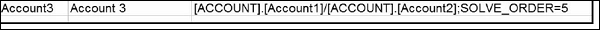This includes movement of calculation dimension formulas from one time period to another.

[AccRec] Accounts Receivable

[AccPay] Accounts Payable

 Mvmt Acc Rec Movement in Accounts Receivable (if([Time].CurrentMember.Children.Count = 0,[Account].[AccRec]-([Account].[AccRec],[Time].PrevMember) SUM(Descendants[Time].CurrentMember,[Time].[Month],LEAVES))) Mvmt inventory Movement in Inventory (if([Time].CurrentMember.Children.Count = 0,[Account].[Inventory]-([Account].[Inventory],[Time].PrevMember) SUM(Descendants[Time].CurrentMember,[Time].[Month],LEAVES)))

Syntax for creating script logic in dimension formulas are already covered.

Exceptions in MDX Syntax

Following are the exceptions to MDX syntax with BPC −

• Replace the keyword AS with an “=” (equal) sign.
• Not to use single quotes around expressions.

Example

[ACCOUNT].[#GROSSSALES] = -[ACCOUNT].[UNITS]*[ACCOUNT].[INPUTPRICE]

[ACCOUNT].[#COST] = -[ACCOUNT].[#GROSSSALES]*80/100

## Worksheet Logic

All the calculations performed in Excel worksheet is known as Worksheet logic. Worksheet logic includes all the functions that are available in an Excel spreadsheet and functions provided in BPC.